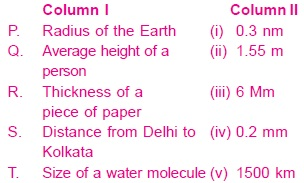# User Forum

Subject :NSO    Class : Class 6

Column II has approximate values of five quantities given in column I. Match column I with column II and select the correct option from the given codes.AP - (v), Q - (iii), R - (i), S - (iv), T - (ii)
BP - (i), Q - (ii), R - (iii), S - (iv), T - (v)
CP - (iii), Q - (ii), R - (iv), S - (v), T - (i)
DP - (iii), Q - (iv), R - (i), S - (v), T - (ii)